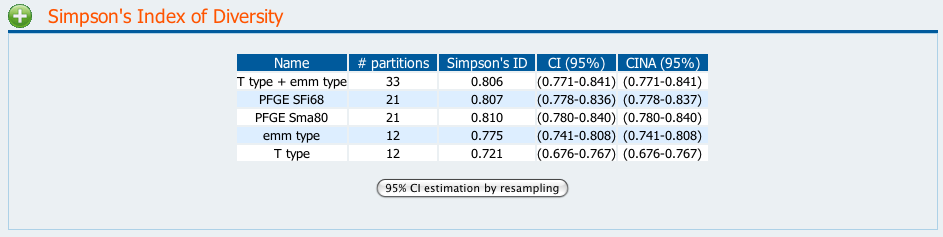Comparing Partitions
Getting started
Diversity
Pairwise agreement
Information theory
Calculations

## Simpson's Index of Diversity

Hunter and Gaston (1988) proposed the use of Simpson's index of diversity (Simpson, 1949) to measure the discriminatory ability of typing systems. This index indicates the probability of two strains sampled randomly from a population belonging to two different types. Grundmann et al. (2001), proposed the use of the large sample approximation for the calculation of confidence intervals for Simpson's index, thereby improving the objective assessment of the discriminatory power of typing techniques. The confidence interval resulting from this approximation (CI) can be compared with the original Non-Approximated Confidence Interval (CINA) described in Simpson (1949).

The formulas for Simpson's index of diversity for a partition A (SIDA) and confidence interval (CI) calculation are:where N is the total sample size, S is the total number of types described (total number of species), ni is the n-th type and pi is the frequency ni /N. According to the first formula, the Simpson's index can assume values from 0 to 1.

When comparing two typing methods, to see which is the more discriminatory, one needs to compare the 95% Confidence intervals: if they overlap one can not exclude the hypothesis that both methods have similar discriminatory power (at a 95% confidence level). In the table presented in Figure 1, by comparing the five typing methods, one can conclude that, for the data set under study, PFGE Sfi68, PFGE Sma80, emm type and the combination of T typing and emm typing, have similar levels of diversity while the characterization by T type results has less diversity than both PFGE methods and the T type/emm type combination. Nevertheless, one can not exclude the hypothesis, at a 95% confidence level, that T type and emm type results having similar Simpson's Index of Diversity values, and therefore similar discriminatory power.Figure 1

It is also possible to estimate a 95% CI by resampling using the jackknife pseudo-values method. After clicking the "95% CI estimation by resampling" button, a new row with the new CI is displayed. A new table with the p-values is also shown. Please see p-values for more information on how to compare results based on p-values.